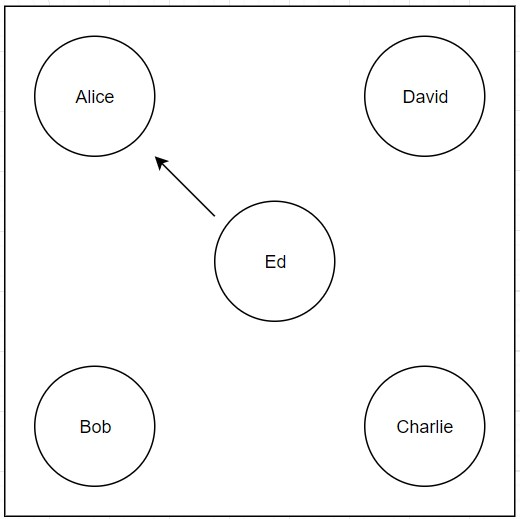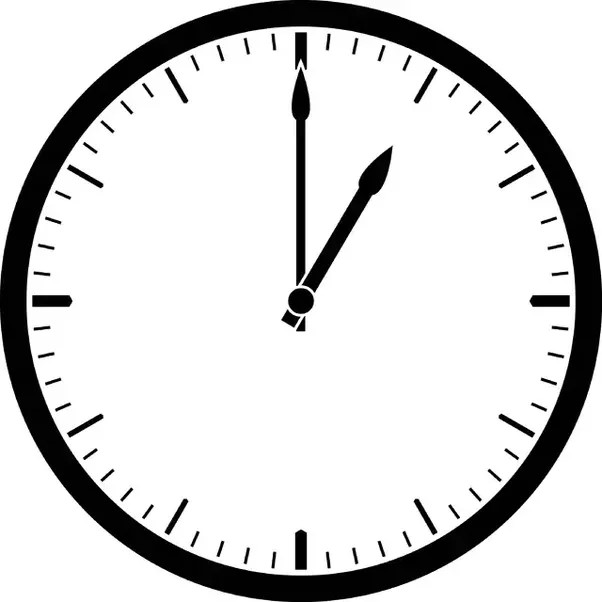# Testing GPT-3 spatial reasoning and comprehension

Marton Trencseni - Sat 17 December 2022 - gpt

## Introduction

In recent months I've played around with OpenAI's GPT-3 and tested it in various domains I work in (have worked in):

My core conclusion in these experiments was that the AI does surprisingly well in these domains, with big caveats: it sounds equally confident in right and wrong answers. But, in retrospect, now that we're used to the idea of GPT-3, perhaps this makes sense, because GPT-3 is trained from Wikipedia articles and other knowledge bases, and a lot of these answer can be — as the system proves — answered just on a statistical pattern-matching / text-completion basis.

What I was wondering recently whether, or to what degree, GPT-3 has developed an understanding of something removed from textual knowledge: spatial reasoning and comprehension. Going into these experiments, I did not know what to expect. GPT-3 has done well in the other domains and surprised me, so my baseline expectation was that I will have a similar experience here: GPT-3 will do surprisingly well.

You can also talk to GPT-3, just create an account on OpenAI and go to the sandbox!

## People in a room

The simplest test I could think of is to place people in 4 corners in a room, put a person in the middle, and ask who is to the left/right/behind the person in the middle. Let's start with an easy question:

Q: There is square room.
Alice is standing in the north-west corner.
Bob is standing in the south-west corner.
Charlie is standing in the south-east corrner.
David is standing in the north-east corner.
Ed is standing in the center of the room, looking at Alice.
How many people are there in the room?

A: Five people are in the room: Alice, Bob, Charlie, David, and Ed.Q: There is square room.
...
Who is standing to the left of Ed?

A: Alice is standing to the left of Ed.

Note: incorrect, Bob is standing to the left of Ed.

Q: There is square room.
...
Who is standing to the right of Ed?

A: Bob is standing to the right of Ed.

Note: incorrect, David is standing to the right of Ed.

Q: There is square room.
...
Who is standing behind Ed?

A: Nobody is standing behind Ed.

Note: incorrect, Charlie is standing behind Ed.

Clearly GPT-3 cannot handle this task. Let's try a variation:

Q: There is square room.
...
Everybody goes around clockwise 90 degrees.
Who is now in the north-west corner?

A: Charlie is now in the north-west corner.

Note: incorrect, Bob is now in the north-west corner.

Perhaps the problem is with using north-south-west-east terminology. Let's simplify:

Q: There is square room.
Alice is standing in the upper left corner.
Bob is standing in the lower left corner.
Charlie is standing in the lower right corner.
David is standing in the upper right corner.
Ed is standing in the center of the room, facing Alice.
Who is standing to the left of Ed?

A: Bob is standing to the left of Ed.

Note: correct!

Q: There is square room.
...
Who is standing to the right of Ed?

A: Charlie is standing to the right of Ed.

Note: incorrect, David is standing to the right of Ed.

Q: There is square room.
...
Who is standing behind Ed?

A: Nobody is standing behind Ed.

Note: incorrect, Charlie is standing behind Ed.

The conlusion is clear: GPT-3 cannot handle this spatial comprehension task.

## Clock faces

Can GPT-3 reason about the hands of a clock?

Q: It is half past eleven. What number is the minute hand of the clock pointing at?

A: The minute hand of the clock is pointing at 30.

Note: correct.

Q: I have a watch. The hour hand is pointing to 11. The minute hand is at a right angle to it, on the right side. What number is the minute hand pointing at?

A: The minute hand is pointing at 2.

Note: correct.

Q: I have a watch. The hour hand is pointing to 11:30. The minute hand is at a right angle to it, on the right side. What number is the minute hand pointing at?

A: The minute hand is pointing at 8.

Note: incorrect, the minute hand is pointing at 2:30.

Let's try the same question, phrased differently:

Q: I have a watch. The hour hand is pointing halfway between 11 and 12. The minute hand is at a right angle to it, on the right side. What number is the minute hand pointing at?

A: The minute hand is pointing to the number 8.

Note: incorrect again.This is a mixed bag. The simpler questions GPT-3 can answer, probably because they occur in flat text it was trained on. But going beyond, it fails.

## Triangles

Q: We have a triangle. One of the angles is 70 degrees, another is 80 degrees. What is the third angle?

A: The third angle is 30 degrees.

Note: correct. This is so simple, it may even have occured with these numbers in the training data. Also, it's known that GPT-3 can do simple arithmetic.

Q: We have a triangle. One of the angles is 70.51 degrees, another is 80.49 degrees. What is the third angle?

A: The third angle is 29 degrees.

Note: correct.

Q: We have two identical right-angled triangles. We snap them together along their longest edges. What is the resulting shape?

A: The resulting shape is a square.

Note: correct.

Q: We have a very small triangle on a very large sphere. What is the sum of its angles, in degrees?

A: 180 degrees

Note: correct, if the triangle is very small, the surface of the sphere is locally flat, so we can approximate with Euclidean geometry.

Q: We have a triangle on a sphere. What is the sum of its angles, in degrees?

A: The sum of the angles of a triangle on a sphere is always 180°.

Note: incorrect. On a sphere, the sum of angles of a triangle is between 180 and 3x90=270 degrees.

Q: We have a triangle on a sphere. The sum of it's angles is 200 degrees. We scale up the sphere by 10x. What is the sum of the triangle's angles now?

A: The sum of the triangle's angles now is 2000 degrees.

Note: incorrect. The angles don't change, the sum remains 200.

This is similar to the clock face thread: GPT-3 falls apart quickly beyond the simplest questions.

## Areas and volumes

Q: We have a white cube, each side is 1 unit long. We paint it red using 1 liter of paint.
We now have a white cube, each side is 2 units long. How many liters of paint do we need to paint it red?

A: We would need 4 liters of paint to paint the cube red. This is because each side of the cube is now twice as long, so the total surface area of the cube has been multiplied by four.

Note: correct.

Q: We have a square. We cut up the square into pieces and rearrange the pieces to form a really long rectangle. Which has bigger area, the original square, or the really long rectangle?

A: The original square has a bigger area than the really long rectangle.

Note: incorrect. Area remains the same.

Q: There is a cube, and a sphere inside the cube. Which has bigger volume?

A: The cube has a larger volume.

Note: correct.

Q: The area of a unit square in 2D is 1, the area of the contained circle is Pi/4.
The volume of a unit cube in 3D is 1, the volume of the contained sphere is Pi/6.
What is the volume of the sphere contained in a unit cube in 4D?

A: The volume of the sphere contained in a unit cube in 4D is 2π/15.

Note: incorrect, the correct answer would be $\frac{\pi^2}{32}$.

Q: In higher dimensions what is the ratio of the volumes of a cube and the sphere contained in the cube??

A: The ratio of the volumes of a cube and the sphere contained in the cube is 8 : π2.

Note: not a good answer. A good answer is that in higher dimensions the ratio if the volume of the contained sphere and the cube goes to zero. In other words, in higher dimensions — against our intuition — the sphere becomes arbitrarily small.

## Random point processes

Can GPT-3 reason about random points in a square?

Q: We have a unit square. We randomly place points within the unit square, each point is independent from the rest, and it's position distribution is uniform in the square. What percent of the points falls within the square?

A: 100%. All points placed within the unit square will fall within the square.

Note: correct.

Q: We have a unit square. We randomly place points within the unit square, each point is independent from the rest, and it's position distribution is uniform in the square. We draw a diagonal from the top left corner to the lower right corner. What percent of points falls below this diagonal?

A: The answer is 50%. Since the points are placed randomly and independently, and the position distribution is uniform, an equal number of points will fall to the left and right of the diagonal line. Therefore, the percentage of points below the diagonal will be 50%.

Note: correct.

Q: We have a unit square. We randomly place points within the unit square, each point is independent from the rest, and it's position distribution is uniform in the square. What percent of points falls within the circle contained in the square?

A: The percentage of points that fall within the circle contained in the square is approximately 78.54%.

Note: correct, $\frac{\pi}{4} = 0.7854$.

To my surprise, after the previous topics, GPT-3 got all these correct. My suspicion is that these questions are close enough to flat text knowledge covered in Wikipedia articles (and similar training documents).

## ASCII art

Can GPT-3 read or write ASCII art? This is the question that originally triggered my exploration into spatial comprehension. Let's see:

Q: What is the letter in the following ASCII art?

██╗░░██╗
██║░░██║
███████║
██╔══██║
██║░░██║
╚═╝░░╚═╝


A: The letter is "E".

Note: incorrect.

Q: What is the text in the following ASCII art?

██╗░░██╗███████╗██╗░░░░░██╗░░░░░░█████╗░
██║░░██║██╔════╝██║░░░░░██║░░░░░██╔══██╗
███████║█████╗░░██║░░░░░██║░░░░░██║░░██║
██╔══██║██╔══╝░░██║░░░░░██║░░░░░██║░░██║
██║░░██║███████╗███████╗███████╗╚█████╔╝
╚═╝░░╚═╝╚══════╝╚══════╝╚══════╝░╚════╝░


A: Welcome!

Note: incorrect, but "Welcome" is eerily close to "Hello". I wonder what's going on here.

Q: What is the text in the following ASCII art?

███╗░░░███╗░█████╗░██████╗░████████╗░█████╗░███╗░░██╗
████╗░████║██╔══██╗██╔══██╗╚══██╔══╝██╔══██╗████╗░██║
██╔████╔██║███████║██████╔╝░░░██║░░░██║░░██║██╔██╗██║
██║╚██╔╝██║██╔══██║██╔══██╗░░░██║░░░██║░░██║██║╚████║
██║░╚═╝░██║██║░░██║██║░░██║░░░██║░░░╚█████╔╝██║░╚███║
╚═╝░░░░░╚═╝╚═╝░░╚═╝╚═╝░░╚═╝░░░╚═╝░░░░╚════╝░╚═╝░░╚══╝


A: No text

Note: incorrect.

Q: Generate ASCII art for the letter H.

A:

  ____
|    |
|    |
|    |
|____|


Note: incorrect.

Q: Generate ASCII art for the word HELLO.

A:

██╗   ██╗██╗ ██████╗ ███████╗    ██╗      ██████╗ ██████╗
██║   ██║██║██╔════╝ ██╔════╝    ██║     ██╔═══██╗██╔══██╗
██║   ██║██║██║  ███╗███████╗    ██║     ██║   ██║██║  ██║
╚██╗ ██╔╝██║██║   ██║╚════██║    ██║     ██║   ██║██║  ██║
╚████╔╝ ██║╚██████╔╝███████║    ███████╗╚██████╔╝██████╔╝
╚═══╝  ╚═╝ ╚════


Note: incorrect, but it's impressive that it's able to generate some ASCII art with letters.

## Conclusion

Based on these experiments, my conlusion is that during its training GPT-3 has not developed the ability to reason spatially. However, based on the recent flow of models released that can paint (DALL-E), code (Codex), chat (ChatGPT), and so on, I assume that given enough training data it would be possible to train models that perform well on spatial reasoning tasks.